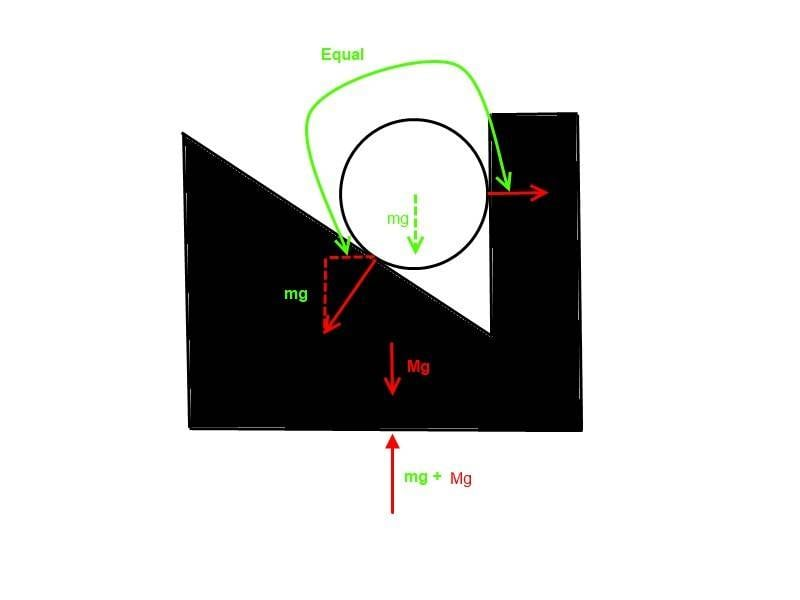# What is the total torque of that system?

Sameh soliman

## Homework Statement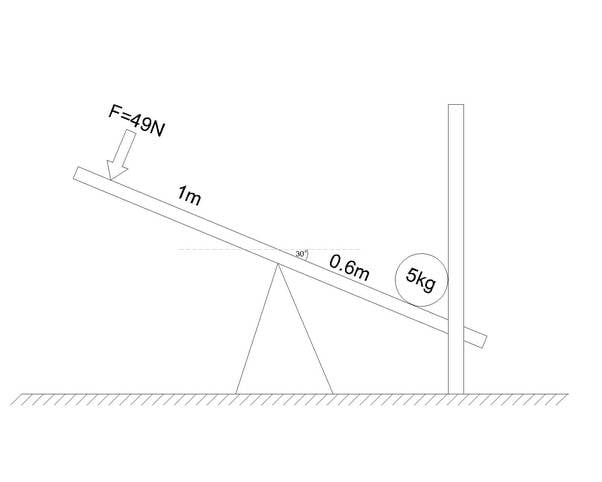A load was put on the right side of a seesaw 0.6 meter from its center as shown, on the other side a force of 49N is applied perpendicular to the seesaw surface 1 meter from its center, the setup is tuned to an angle of 30 from the horizontal axis as shown.

On the right side there is a wall perpendicular to the ground surface and overlap the seesaw but having a gap in the middle to allow the seesaw to path throw it easily while the load is wider than the seesaw so that it can interact with the wall.

Neglecting all frictions state the following.

a) State if or if not the seesaw will be able to move and why.

b) State the total torque of the system.at its initial start

t = r x F[/B]

## The Attempt at a Solution

Below is my solution but i am not sure if it was right !!

-First i assume the wall on the right side is not there

torque produced by the left side = 1m X 49N =49Nm ANTICLOCKWISE .
torque produced on the right side is due to the vector force of gravity but only the sub-vector perpendicular to the seesaw surface which i figured that it will be =
cos30 X 5kg X 9.8m2 = 42.44N
so the torque on the right side = 0.6m x 42.44N = 25.46Nm CLOCKWISE

So the total torque of the system if the wall was not there will be =
49Nm - 25.46Nm = 23.54Nm ANTICLOCKWISE

-Now i study the interaction between the system and the wall

As the seesaw trying to rotate anticlockwise the object on the right will exert a force on the wall and since the total torque of the system is 23.54Nm so the total force the object can produce is =
F = t/r = 23.54Nm / 0.6 m = 39.23 Nm in a direction that is perpendicular to the seesaw surface .
but since there is no friction so the only force that will affect the wall is the sub-vector perpendicular to the wall surface which i figured out will be equal to =
cos60 x 39.23N = 19.62N

And since the wall is attached to the ground and will not move so the wall will exert a normal force on the system in the negative direction but only the sub vector that is perpendicular to the seesaw surface will affect the torque and i figured out that that sub vector =
cos60 x 19.62N = 9.81N in the negative direction

so the torque that this normal force will produce on the system =
0.6m x 9.81N = 5.89Nm CLOCKWISE

SO THE TOTAL TORQUE OF THE SYSTEM AT ITS INITIAL STAR WILL BE =
23.54Nm - 5.89Nm = 17.65Nm
AND SINCE THERE IS NO FRICTION SO THE SYSTEM WILL MOVE ANTICLOCKWISE WHILE THE OBJECT ON THE LEFT WILL SLIDE TOWARD THE CENTRE OF THE SEESAW

[/B]

#### Attachments

Last edited:

Homework Helper
Gold Member
but since there is no friction so the only force that will affect the wall is the sub-vector perpendicular to the wall surface which i figured out will be equal to =
cos60 x 39.23N = 19.62N

so the torque that this normal force will produce on the system =
0.6m x 9.81N = 5.89Nm CLOCKWISE

To calculate the torque generated by the normal force of the wall you'd need the geometry of the load to find the lever. Could you elaborate on how you've calculated it? Did you draw a FBD of the load?

Sameh soliman
To calculate the torque generated by the normal force of the wall you'd need the geometry of the load to find the lever. Could you elaborate on how you've calculated it? Did you draw a FBD of the load?

Yes, I have drawn the FBD diagram but i assumed all forces acts on the point where the object interact with the seesaw.

But it was given that the object is cylindrical with radius of 0.15 m but i wasn't sure what to do with it and i didn't include it in the details

Homework Helper
Gold Member
Yes, I have drawn the FBD diagram but i assumed all forces acts on the point where the object interact with the seesaw.

In the attached schematic, assume the load is fixed (by glue or screw etc.) to the see saw. Which force would you use to calculate the torque generated by the load?

#### Attachments

Sameh soliman
In order for the seesaw to move anticlockwise in presence of the wall, then it have to break the force that attache the object to it.
so i think force A will be vital in deciding if the seesaw will move or not because if it exceed the torque the seesaw will not move at all.

Homework Helper
Gold Member
In order for the seesaw to move anticlockwise in presence of the wall, then it have to break the force that attache the object to it.
so i think force A will be vital in deciding if the seesaw will move or not because if it exceed the torque the seesaw will not move at all.

Same scenario with the load fixed and without wall to the seesaw: Which of the two drawings you think will be the final position?

#### Attachments

Sameh soliman
I think you are assuming that there is no force on the other side of the seesaw, so in that case the figure on the right will be right because the object centre of gravity will be directly under the centre of the seesaw

Homework Helper
Gold Member
I'm in a rush so not sure if this helps but...

If there is zero friction between two objects that usually tells you something about the direction of forces between them.

Sameh soliman
Same scenario with the load fixed and without wall to the seesaw: Which of the two drawings you think will be the final position?

Starting to get what you mean

you mean the forces should be calculated as acting on the centre of the object

Homework Helper
Gold Member
Starting to get what you mean

you mean the forces should be calculated as acting on the centre of the object

Yes. So regarding your first calculation (without wall and the load not sliding, because it is attached to seesaw somehow), you have to take into account the increase of the lever due to the inclination, since the force is "shifted upwards" by the radius of the load.

Sameh soliman
Yes. So regarding your first calculation (without wall and the load not sliding, because it is attached to seesaw somehow), you have to take into account the increase of the lever due to the inclination, since the force is "shifted upwards" by the radius of the load.
so using the radius for the circle the angle of inclination is 14 degrees, so i should subtract that from the given angle which is 30 degrees when calculating the torque on the right side produced by gravity ..... right ?

also use the new angle in calculating the sub-vector of the normal force that will affect the torque.....right ?

also please state if there was any thing else i am missing and thank you so much for your help.

Homework Helper
Gold Member
so using the radius for the circle the angle of inclination is 14 degrees, so i should subtract that from the given angle which is 30 degrees when calculating the torque on the right side produced by gravity ..... right ?

I think it would be easier to calculate the distance ##d'## as indicated in the image attached. You just need to know the value of ##\theta##.

also use the new angle in calculating the sub-vector of the normal force that will affect the torque.....right ?

Unfortunately no. If the radius would be zero (so the mass could be modelled as point), then you could use the normal force to calculate the torque of the weight. If you have a look at the picture in the post #6 (left-hand side)): Could you calculate the torque generated by the weight using the reaction forces?

#### Attachments

Sameh soliman
I think it would be easier to calculate the distance ##d'## as indicated in the image attached. You just need to know the value of ##\theta##.

Unfortunately no. If the radius would be zero (so the mass could be modelled as point), then you could use the normal force to calculate the torque of the weight. If you have a look at the picture in the post #6 (left-hand side)): Could you calculate the torque generated by the weight using the reaction forces?

yes ... if the object was also a cylinder with 0.15m radius that have mass of 5kg then the force affecting the torque will be the sub-vector of gravity perpendicular to the line between the centre of the seesaw and the centre of the object = cos76 x 5kg x 9.8m2 = 11.85 N

t = 0.62m x 11.84N = 7.34Nm

0.62 is the length between the centre of the seesaw and the centre of the object

Hope that i am right ...... but i am sorry i still cant understand how this is related to the effect of the normal force

Last edited:
Homework Helper
Gold Member
yes ... if the object was also a cylinder with 0.15m radius that have mass of 5kg then the force affecting the torque will be the sub-vector of gravity perpendicular to the line between the centre of the seesaw and the centre of the object = cos76 x 5kg x 9.8m2 = 11.85 N

Sorry, I got lost. But what I was thinking about was (by the way ##\alpha=\theta##):

$$l=\left(d+\underbrace{r\;tan\alpha}_{d'}\right)cos\alpha \approx 0.59$$
$$mgl =29.17\;Nm$$

Homework Helper
Gold Member
I drew a FBD of the cylinder and calculated the clockwise torque as follows...

The normal force on the cylinder from the beam is mg/cos(30) at 0.6m from the pivot. That works out at 34Nm.

The anticlockwise torque is 49Nm so I believe it rotates anticlockwise.

Sameh soliman
Sorry, I got lost. But what I was thinking about was (by the way ##\alpha=\theta##):

$$l=\left(d+\underbrace{r\;tan\alpha}_{d'}\right)cos\alpha \approx 0.59$$
$$mgl =29.17\;Nm$$
Sorry ..... but i am really very lost now

Sameh soliman
I drew a FBD of the cylinder and calculated the clockwise torque as follows...

The normal force on the cylinder from the beam is mg/cos(30) at 0.6m from the pivot. That works out at 34Nm.

The anticlockwise torque is 49Nm so I believe it rotates anticlockwise.
Can you please clarify more ..... because according to my previous calculations the normal force should be much more than that you mentioned.

Homework Helper
Gold Member
Can you please clarify more ..... because according to my previous calculations the normal force should be much more than that you mentioned.

Not too much time today, sorry (compare the symbols with the schematic):

Scenario 1 (without wall, the ball attached somehow to the seesaw - you didn't mentioned that in your first post, but I assumed it):

$$l=\left(d+\underbrace{r\;tan\alpha}_{d'}\right)cos\alpha \approx 0.59$$
$$mgl =29.17\;Nm$$

$$N=mg cos\alpha$$
$$Nd = 25.49\;Nm$$

$$\rightarrow mgl \neq Nd$$

If the radius ##r## of the load would be zero, the two expressions would be equal.

Scenario 2 (with wall):

$$l=\left(d+\underbrace{r\;tan\alpha}_{d'}\right)cos\;\alpha \approx 0.59$$
$$mgl =29.17\;Nm$$

$$W=mg\;tan\;\alpha$$
$$h = \left(d+d'\right)sin\;\alpha-\frac{r}{cos\alpha}\approx 0.17\;m$$
$$Wh\approx 4.81\;Nm$$

$$Wh+mgl=34\;Nm$$

$$N=\frac{mg} {cos\alpha}$$
$$Nd=34\;Nm$$

$$\rightarrow mgl+Wh = Nd$$

Since the "counterforce" of the wall increases the normal force, the results obtained with the two calculations are identical.

#### Attachments

•Sameh soliman
Homework Helper
Gold Member
Can you please clarify more ..... because according to my previous calculations the normal force should be much more than that you mentioned.

OK here is my working. This is the FBD I did for the cylinder...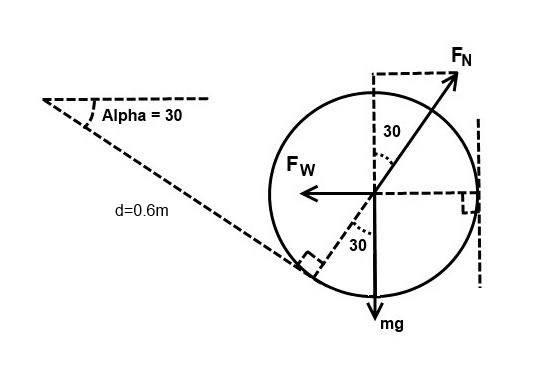mg is the weight of the cylinder
Fw is the normal force at the wall
FN is the normal force with the plank

Fw and FN must be perpendicular to the wall/plank because there is no friction at either point.

If the cylinder isn't accelerating then the vertical force must sum to zero. So..

FN*Cos(30) = mg
then
FN = mg/cos(30)

So the clockwise torque is d*FN = dmg/cos(30) = 0.6*5*9.81/cos(30) = 29.43/cos(30) = 33.98Nm

The anticlockwise torque is just 49*1=49nM.

There is a net anticlockwise torque of 49 - 34 = 15Nm

Have I missed something?

#### Attachments

Last edited:
•Sameh soliman
Homework Helper
Gold Member
Not sure I follow what stockzahn is saying but I also made this drawing that shows that Fw is effectively already included in FN. …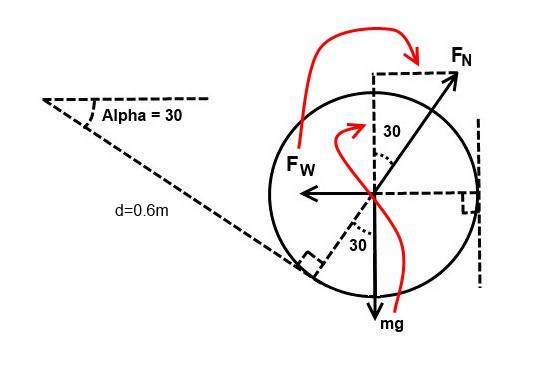So Fw = MgTan(30) = FnSin(30)

#### Attachments

Homework Helper
Gold Member
And since the wall is attached to the ground and will not move so the wall will exert a normal force on the system....

I think this is why you are confused. It exerts a force on the cylinder NOT the system.

You should read up on free body diagrams and how they should be used. Mine might not be perfect.

The key is that you should draw a _separate_ FBD for each part of the system. Eg One for the plank. A separate one for the cylinder etc. Perhaps a third for the wall if necessary. Then each FBD should only show forces that act on that body. No others.

If you did this it would be clear that only the normal force acts between the cylinder and plank.

The force between the wall and the cylinder only acts on the cylinder not the plank or the "system".

•Sameh soliman
Homework Helper
Gold Member
Not sure I follow what stockzahn is saying but I also made this drawing that shows that Fw is effectively already included in FN. …

I just wanted to point out that in the first scenario of the OP's first post one cannot solve this problem only using the normal force.

Sameh soliman
OK here is my working. This is the FBD I did for the cylinder...

View attachment 230900

mg is the weight of the cylinder
Fw is the normal force at the wall
FN is the normal force with the plank

Fw and FN must be perpendicular to the wall/plank because there is no friction at either point.

If the cylinder isn't accelerating then the vertical force must sum to zero. So..

FN*Cos(30) = mg
then
FN = mg/cos(30)

So the clockwise torque is d*FN = dmg/cos(30) = 0.6*5*9.81/cos(30) = 29.43/cos(30) = 33.98Nm

The anticlockwise torque is just 49*1=49nM.

There is a net anticlockwise torque of 49 - 34 = 15Nm

Have I missed something?
THAT DID IT ...... Thanks

Sameh soliman
Not sure I follow what stockzahn is saying but I also made this drawing that shows that Fw is effectively already included in FN. …
View attachment 230906

So Fw = MgTan(30) = FnSin(30)
Thanks so much for your help..... but i have a question .

you said that since the object isn't moving vertically then FN*Cos(30) = mg, which mean that the total magnitude of Fn is greater than mg.
so my question is ... Since mg is the only exterior force acting on the object then how could a magnitude of a counter force exceed it?

Homework Helper
Gold Member
We aren't calculating Fn produced as a result of mg.

What we are calculating is the force Fn required to hold it still. This calculated value is then compare with the torque provided by the 49N force the other end. Since one is greater than the other it's not still but accelerating.

Imagine if the plank was at a much greater angle, say 89 degrees, nearly vertical. With no friction the beam would have to press the cylinder very hard against the wall to stop the cylinder falling.

If the angle was zero, horizontal, the beam would only have to provide Fn=mg to stop the cylinder falling.

Last edited:
Homework Helper
Gold Member
If the cylinder was stationary Fn could still be greater than mg. The cylinder is acting like a wedge between the plank and the wall. The force required to drive a wedge between two surfaces is less than the force pressing them together (ignoring friction).

Sameh soliman
We aren't calculating Fn produced as a result of mg.

What we are calculating is the force Fn required to hold it still. This calculated value is then compare with the torque provided by the 49N force the other end. Since one is greater than the other it's not still but accelerating.

Imagine if the plank was at a much greater angle, say 89 degrees, nearly vertical. With no friction the beam would have to press the cylinder very hard against the wall to stop the cylinder falling.

If the angle was zero, horizontal, the beam would only have to provide Fn=mg to stop the cylinder falling.
Ok ..... to make it more clear for me let's imagine something else .
The same last diagram you drawn but this time there is no seesaw but it is an object on a ramp that have the same angle we used, this ramp has a vertical wall attached to it in which the object is stuck infront of it.

Also there is no friction.

Will the same calculations we previously used appy?

Homework Helper
Gold Member
The same last diagram you drawn but this time there is no seesaw but it is an object on a ramp that have the same angle we used, this ramp has a vertical wall attached to it in which the object is stuck infront of it.

Also there is no friction.

Will the same calculations we previously used appy?

Yes...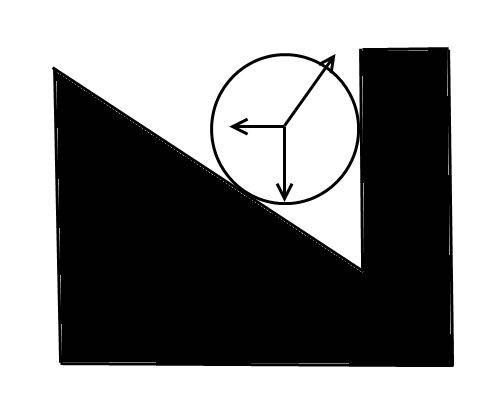Since mg is the only exterior force acting on the object then how could a magnitude of a counter force exceed it?

If this is still difficult to understand think about the tension in a tightrope. The tension must far exceed the weight of the man balancing on it. Indeed if you try and make the tightrope perfectly horizontal the tension required is infinite.

Scroll down..
https://www.quizover.com/physics/test/what-is-the-tension-in-a-tightrope-by-openstax

#### Attachments